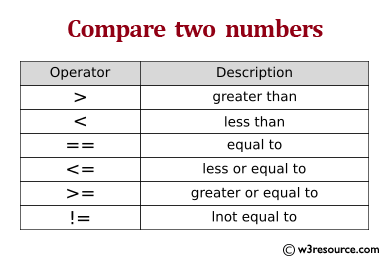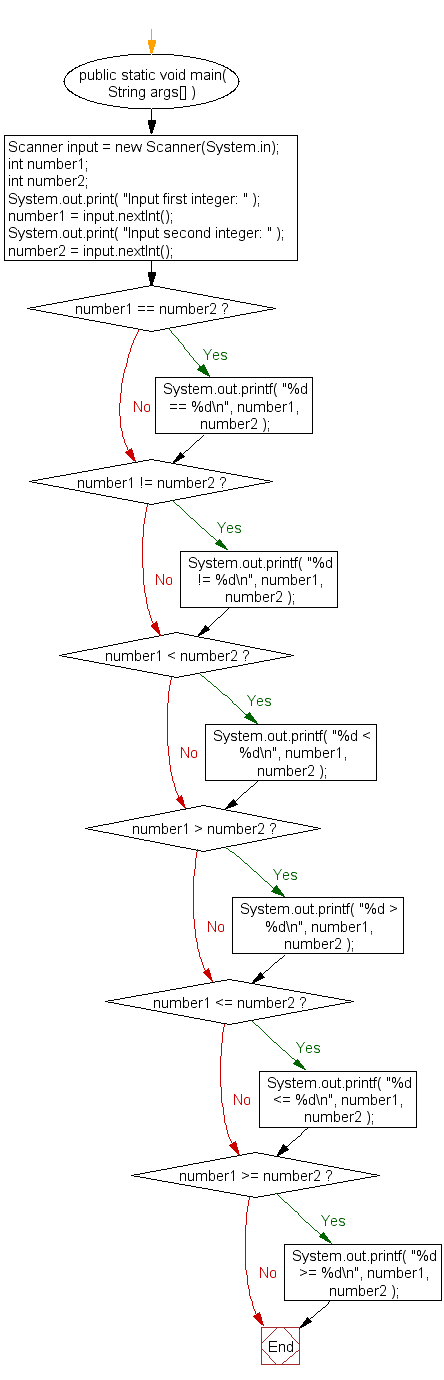﻿ Java exercises: Compare two numbers - w3resource# Java Exercises: Compare two numbers

## Java Basic: Exercise-32 with Solution

Write a Java program to compare two numbers.

Test Data:
Input first integer: 25
Input second integer: 39

Pictorial Presentation:Sample Solution:

Java Code:

``````import java.util.Scanner;
public class Exercise32 {
public static void main( String args[] )
{
// create Scanner to obtain input from command window
Scanner input = new Scanner(System.in);
int number1; // first number to compare
int number2; // second number to compare

System.out.print( "Input first integer: " ); // prompt
number1 = input.nextInt(); // read first number from user

System.out.print( "Input second integer: " ); // prompt
number2 = input.nextInt(); // read second number from user

if ( number1 == number2 )
System.out.printf( "%d == %d\n", number1, number2 );
if ( number1 != number2 )
System.out.printf( "%d != %d\n", number1, number2 );
if ( number1 < number2 )
System.out.printf( "%d < %d\n", number1, number2 );
if ( number1 > number2 )
System.out.printf( "%d > %d\n", number1, number2 );
if ( number1 <= number2 )
System.out.printf( "%d <= %d\n", number1, number2 );
if ( number1 >= number2 )
System.out.printf( "%d >= %d\n", number1, number2 );
}
}
```
```

Sample Output:

```Input first integer: 25
Input second integer: 39
25 != 39
25 < 39
25 <= 39
```

Flowchart:Java Code Editor:

What is the difficulty level of this exercise?

Test your Programming skills with w3resource's quiz.

﻿

## Java: Tips of the Day

countOccurrences

Counts the occurrences of a value in an array.

Use Arrays.stream().filter().count() to count total number of values that equals the specified value.

```public static long countOccurrences(int[] numbers, int value) {
return Arrays.stream(numbers)
.filter(number -> number == value)
.count();
}
```

Ref: https://bit.ly/3kCAgLb